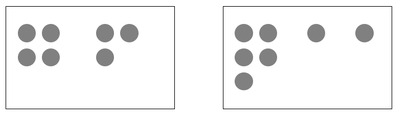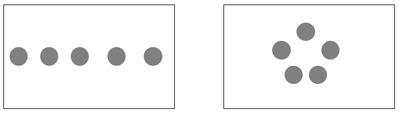# Equality Number Sentences

Alignments to Content Standards: 1.OA.D.7

Compare the number of circles in each box. If they are equal, write a number sentence. For example:$$4+3=5+1+1$$

If they are not equal, write "not equal."

1.2.3.4.5.6.## IM Commentary

The purpose of this instructional task is for students to help students understand the meaning of the equal sign and to use it appropriately. The idea is that students should be comparing the number of circles in each of the rectangles and to write an equation that reflects the fact there are an equal number in each of the boxes (when this is the case). The teacher may need to provide additional explanation to help students understand exactly how the number sentences are intended to represent the pictures. For example, if the groupings indicate an equality such as "2 + 5 = 3 + 4", then the answers "7" or "7 + 7 = 14" are not representing this equality.

Some students might come up with alternate number sentences than those shown in the solution. These should be recognized as correct, but then the teacher can say for example, "I see a group of two and another group of three in that box so in my number sentence I would describe that as 2+3."

This standard is based on research into how students understand the equals sign. Here are some links to further reading:

http://ncisla.wceruw.org/publications/articles/AlgebraNCTM.pdf

http://sddial.k12.sd.us/esa/grants/sdcounts/sdcounts08-09/fall08/Equal_Sign.pdf

## Solution

1. $3 + 2 + 1 = 3 + 3$
2. Not Equal
3. $5 + 3 = 8$
4. $2 + 2 + 2 = 3 + 3$
5. Not Equal
6. $1 + 1 + 1 + 1 + 1 = 5$